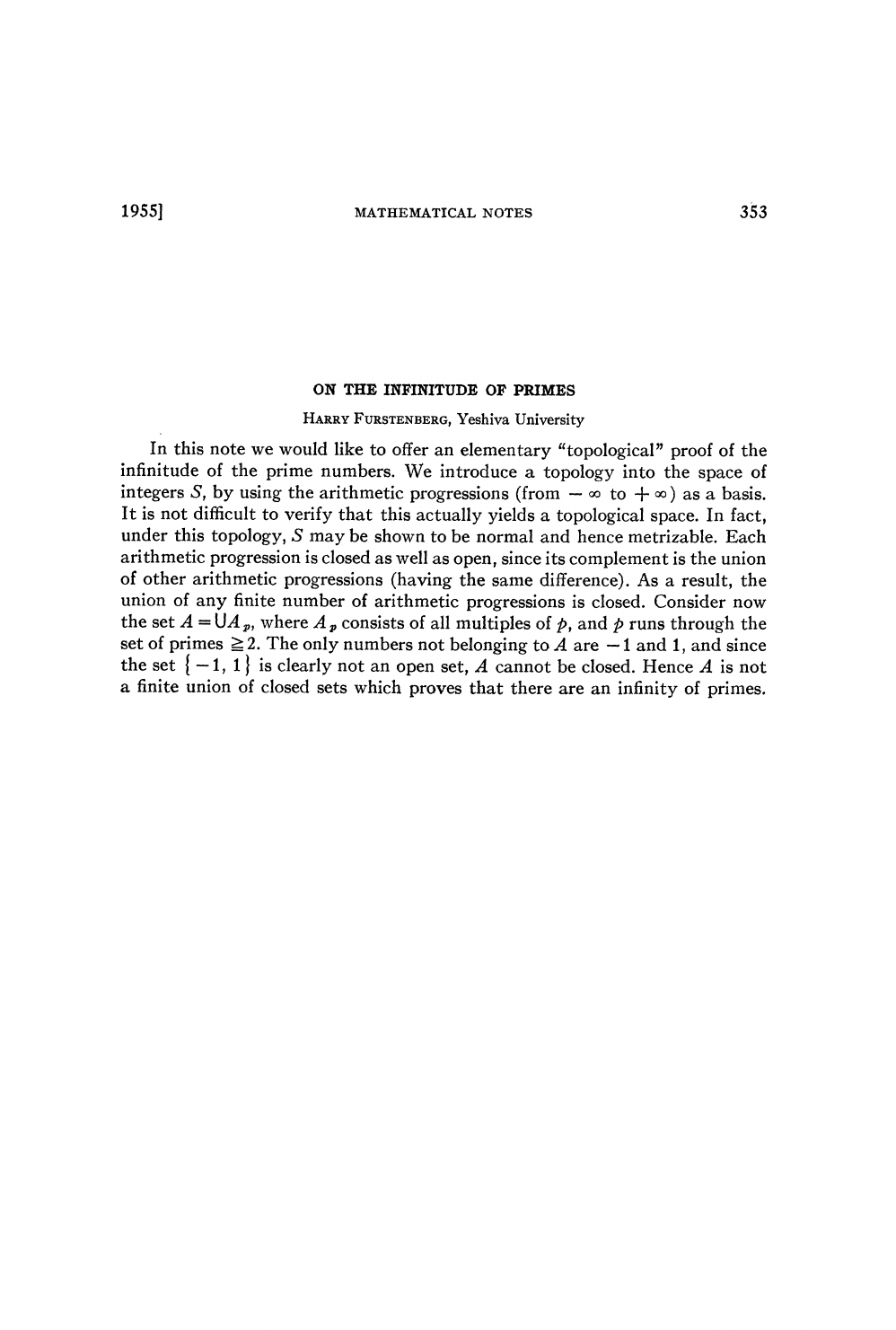Harry Furstenberg is an American-Israeli mathematician and a laurea...
This proof is featured in "Proofs from the Book" by Martin Aigner, ...
A basis for a topology on $\mathbb{Z}$ is a collection B of subsets...
The author is defining a subset U of Z to be open if for every $m \... We say a set is closed if its complement is open. It's not difficul...We say a set is closed if its complement is open. It's not difficult to see that the complement of$N_{7,3}$is$N_{8,3} \cup N_{9,3}$. By definition since$N_{7,3}$is open its complement is closed but at the same time by axiom #2 we have that$N_{8,3} \cup N_{9,3}$is open -$N_{a,b}$is open and closed at the same time! How can$\mathbb{Z}$be$N_{1,0}$if$b > 0$by definition? Is it$N_{1,1}$? Harry Furstenberg is an American-Israeli mathematician and a laureate of the Wolf Prize in Mathematics. In 1955, when Harry was still a 20 years-old undergraduate student at Yeshiva University he gained attention for coming up with this innovative topological proof of the infinitude of prime numbers. ![](https://upload.wikimedia.org/wikipedia/commons/a/a7/Harry_Furstenberg.jpeg) This proof is featured in "Proofs from the Book" by Martin Aigner, Günter M. Ziegler. This book is supposed to present some of the most elegant proofs. Its title is a reference to a story by Erdos about a book where God has written the most beautiful proofs. Yes, that's a typo :) -$N_{1,1}$A basis for a topology on$\mathbb{Z}$is a collection B of subsets of$\mathbb{Z}$(called basis elements) satisfying the following properties. 1. For each x in$\mathbb{Z}$, there is at least one basis element B containing x. 2. If x belongs to the intersection of two basis elements$B_1$and$B_2$, then there is a basis element$B_3$containing x such that$B_3 \subset B_1 \cap B_2$. It is not difficult to see that 1. is verified because for any x in$\mathbb{Z}$, we can always have arithmetic progressions that include x. To prove 2. let's suppose$a_{1}$and$a_{2}$are the differences between successive terms in the sequences$B_{1}$and$B_{2}$. If$a$is the least common multiple of$a_{1}$and$a_{2}$, then$B_{3}=\{\ldots,n-a, n, n+a, n+2a,\ldots\}$. We have now proven that the sequence of arithmetic progressions forms a basis for a topology on$\mathbb{Z}$! The author is defining a subset U of Z to be open if for every$m \in U$, there is a set of the form $$N_{a,b} = \{a+nb: n \in \mathbb{Z}\}, \ \ b>0$$ such that$m \in N_{a,b} \subset U$. Here's an example of what$N_{1,2}$looks like ![](https://i.imgur.com/6tv2viq.png) Now that we've defined our open sets, we need to make sure they satisfy the axioms of topology: #1 both the empty set and$\mathbb{Z}$are open #2 a union of open sets is also open #3 a finite intersection of open sets is open For axiom #1, by definition, the empty set is open;$\mathbb{Z}$is just the sequence$N_{1,0}$, and so is open as well. For axiom #2, it's not difficult to see that$N_{a,b} \cap N_{c,d} = N_{a+c,b+d}$For axiom #3, we note that$N_{a,b} \cup N_{a,c} = N_{a,lcm(b,c)}$where$lcm(b,c)\$ is the least common multiple of b and c.